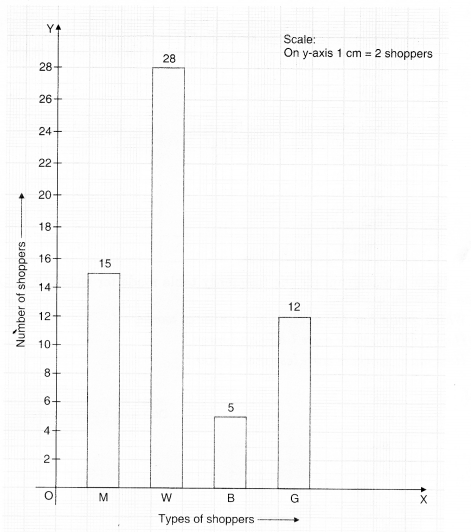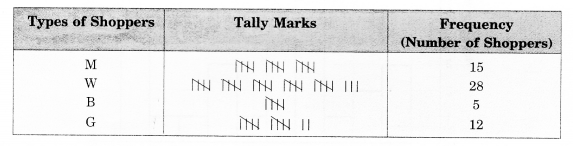NCERT Solutions for Class 8 Maths Chapter 5 Data Handling Ex 5.1 are part of NCERT Solutions for Class 8 Maths. Here we have given NCERT Solutions for Class 8 Maths Chapter 5 Data Handling Ex 5.1.

 Board CBSE Textbook NCERT Class Class 8 Subject Maths Chapter Chapter 5 Chapter Name Data Handling Exercise Ex 5.1 Number of Questions Solved 5 Category NCERT Solutions

## NCERT Solutions for Class 8 Maths Chapter 5 Data Handling Ex 5.1

Question 1.
For which of these would you use a histogram to show the data?
(a) The number of letters for different areas in a postman’s bag.
(b) The height of competitors in an athletics meet.
(c) The number of cassettes produced by 5 companies.
(d) The number of passengers boarding trains from 7:00 a.m. to 7:00 p.m at a station.
Give reasons for each.
Solution.
For (b) and (d) because in these two cases data can be grouped into class intervals.

Question 2.
The shoppers who come to a departmental store are marked as man (M), woman (W), boy (B) or girl (G). The following list gives the shoppers who came during the first hour in the morning.
WWWGBWWMGGMMWWWWG
BMWBGGMWWMMWW
WMWBWGMWWWWGWMMWW
MWGWMGWMMBGGW
Make a frequency distribution table using tally marks. Draw a bar graph to illustrate it.
Solution.Question 3.
The weekly wages (in of 30 workers in a factory are:
830, 835, 890, 810, 835, 836, 869, 845, 898, 890, 820, 860,
832, 833, 855, 845, 804, 808, 812, 840, 885, 835, 835, 836,
878, 840, 868, 890, 806, 840.
Solution.Question 4.
Draw a histogram for the frequency table made for the data in Question 3, and answer the following questions:
(i) Which group has the maximum number of workers?
(ii) How many workers earnt 850 and more?
(iii) How many workers earn less than? 850?
Solution.(i) Group 830—840, has the maximum number of workers.
(ii) 1 + 3 + 1 + 1 + 4 = 10, workers earn ? 850 and more.
(iii) 3 + 2 + l + 9 + 5 = 20, workers earn less than ? 850.

Question 5.
The number of hours for which students of a particular class watched television during holidays is shown through the given graph: